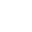• FAQ
• Contact/ Homework Answers / Physics / Question Five possible transitions for a hydrogen atom listed below: Select
Not my Question
Flag Content

# Question : Question Five possible transitions for a hydrogen atom listed below: SelectQuestion

Five possible transitions for a hydrogen atom are listed below: Select whether the atom gains or loses energy for each transition.

Loses: ni = 7; nf = 3
Loses: ni = 7; nf = 3
Gains: ni = 2; nf = 5
Gains: ni = 3; nf = 4
Gains: ni = 4; nf = 6

Find the transition where the atom gains the most energy. How much energy does the atom gain?

Find the transition which will emit the shortest wavelength photon. What is the energy of the photon of this transition?

## Solution 5 (1 Ratings )

Solved
Physics 4 Months Ago 7 Views